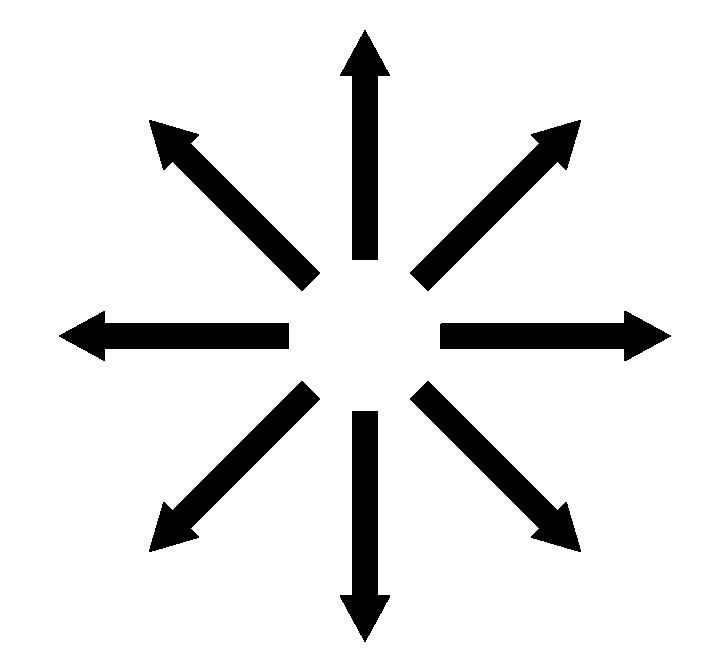#### Howdy, Stranger!

It looks like you're new here. If you want to get involved, click one of these buttons!

Supported by

# Problem with element clicked for arrows

Hello,

I am implementing a rotation span task and here is the thing.

I have a canvas of arrow in different direction appearing on the screen, during a test part, in which the task of the participant ti to click on the arrow previously presented in a correct order.
Anyway the issue I have right now is that when I try to collect where participants click, I have very odd results. For some reasons, even if they click between arrows, then element will take a value that is close to an arrow. My arrow are black on a white screen this way:The code I use to generate them is as follow:

``````import numpy as np

# calculate length total of an arrow,its position at the beginningm and its position at the end
# relatively to the height of the screen
def calc_parameter_arrow(prop_length_arrow, prop_start_point_arrow):
length_total = int(prop_length_arrow*var.height)
pos_arrow_begin = int(prop_start_point_arrow*var.height)
pos_arrow_final_long = length_total + pos_arrow_begin
return (length_total, pos_arrow_begin, pos_arrow_final_long)

# convert polar to cartesian
def pol2cart(rho, phi):
x = rho * np.cos(phi)
y = rho * np.sin(phi)
return(int(round(x)), int(round(y)))

# transform a length according to various radian, and return a list of list with the x, y cartesian coordinate of this length for these various radians

cartesian_pos_return = [pol2cart(p, p) for p in deg_rad_pos]
return(cartesian_pos_return)

#calculate radian of arrow separated by 45 degrees
nbr_arrow = 8.
degree_sep = 45.
deg = np.arange(nbr_arrow) * degree_sep
var.deg_name = [ int(x) for x in deg ]

# various proportion of arrow relative to the height of the screen
var.prop_arrow_long_length_height = 0.3
var.prop_start_point_arrow_long = 0.1

length_arrow_long_total, pos_arrow_begin_long, pos_arrow_final_long = calc_parameter_arrow(var.prop_arrow_long_length_height, var.prop_start_point_arrow_long)

Pratice_arrow_long_Instruct_canvas = Canvas()

for pos, (coord_cart_b, coord_cart_f) in enumerate(zip(cartesian_pos_arrow_begin_long, cartesian_pos_arrow_final_long)):

Pratice_arrow_long_Instruct_canvas['long_arrow%s' % int(var.deg_name[pos])] = Arrow(coord_cart_b,coord_cart_b, coord_cart_f, coord_cart_f, head_width = 50, body_length = 0.8, body_width = 0.5, fill = True, color = 'black')
``````

And in order to lauch the canvas and see my clicked element in the variable inspector:

``````my_mouse = Mouse(visible=True)
counter = -1
Pratice_arrow_long_Instruct_canvas.show()

while counter <15:
button,  (x, y), timestamp = my_mouse.get_click()
var.element_clicked = Pratice_arrow_long_Instruct_canvas.elements_at(x, y)
Pratice_arrow_long_Instruct_canvas.show()
``````

And when I click between the arrows, for some reason var.element_clicked is taking the value of closest arrow.
I do not really know what I did wrong.

Thank you for any help I also join an example script.

Best,

Sylvain

• Oh actually I think I noticed something.
The issue is only happening for arrows that are not in a vertical or horizontal direction.
Like if all the pixels in a square around the arrows were considered as belonging to it.

• Hi Sylvain,

Right now the `Canvas.elements_at()` indeed checks whether a point falls inside the bounding rectangle of a polygon. Thinking about how to improve this I came across `shapely` which seems like a good library for this kind of geometrical operations. If you add the following code to the start of the experiment, the arrow and polygons should accurately indicate whether or not a point falls inside them. (This monkey patches the `Polygon` class.)

Could you let me know if this works for you?

Cheers,
Sebastiaan

``````from openexp._canvas._polygon.polygon import Polygon
from shapely.geometry import Polygon as ShapelyPolygon, Point

def polygon_contains(self, xy):

if not hasattr(self, '_shapely_polygon'):
self._shapely_polygon = ShapelyPolygon(self.vertices)
return self._shapely_polygon.contains(Point(*xy))

def polygon_on_attribute_change(self, **kwargs):

if hasattr(self, '_shapely_polygon'):
del self._shapely_polygon

Polygon.__contains__ = polygon_contains
Polygon.on_attribute_change = polygon_on_attribute_change
``````
• Hey Sebastiaan,